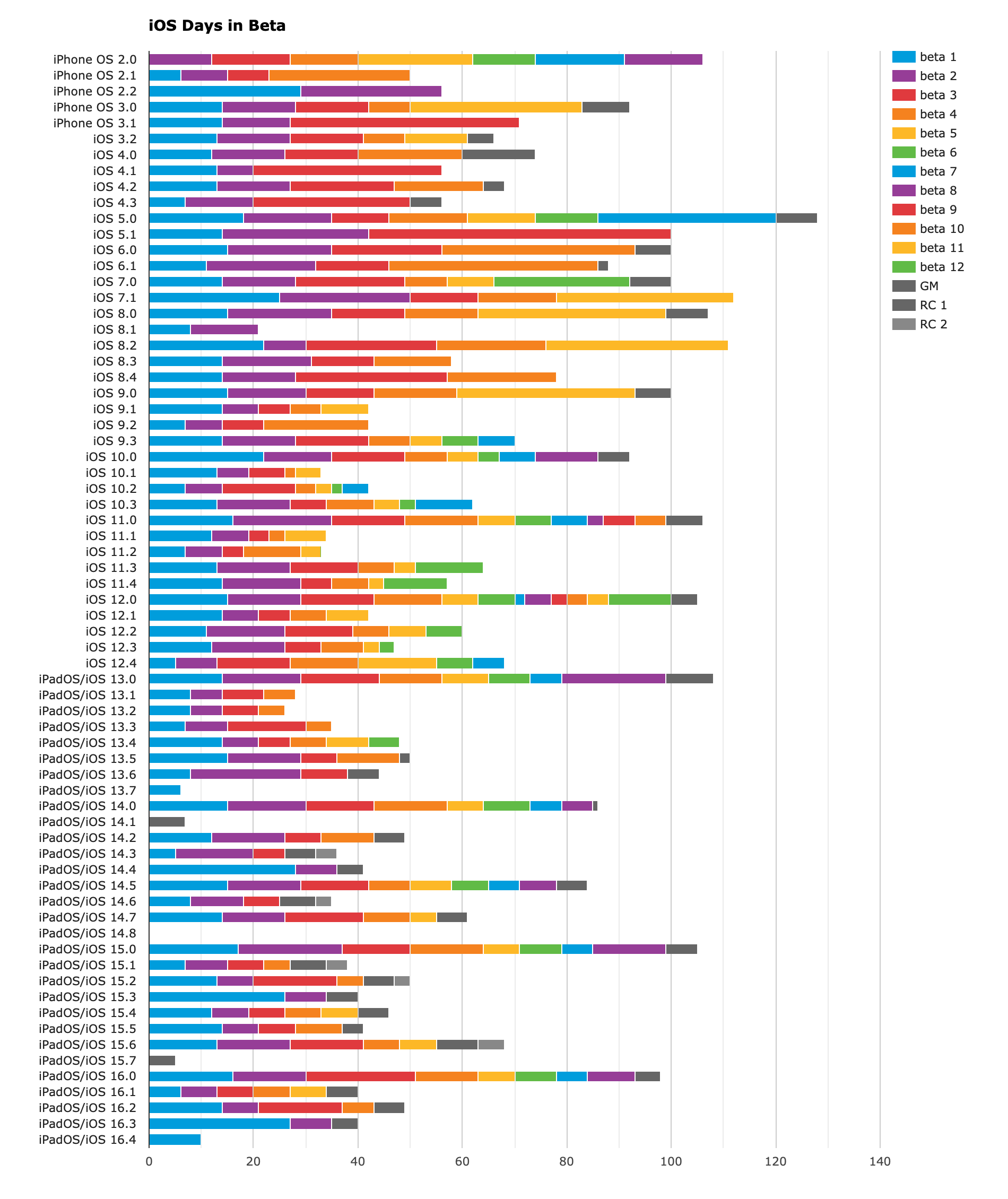ios

# iOS version release date historyself.__wrap_b=(e,n,t)=>{t=t||document.querySelector(`[data-br="\${e}"]`);let c=t.parentElement,r=v=>t.style.maxWidth=v+"px";t.style.maxWidth="";let o=c.clientWidth,l=c.clientHeight,a=o/2,i=o,d;if(o){for(;a+1<i;)d=~~((a+i)/2),r(d),c.clientHeight===l?i=d:a=d;r(i*n+o*(1-n))}t.__wrap_o||(t.__wrap_o=new ResizeObserver(()=>{self.__wrap_b(0,+t.dataset.brr,t)})).observe(c)};self.__wrap_b(":R25d6:",1)

Curious to know about iOS release date versions? Will Hains ↗︎ maintains this helpful (and accessible) chart ↗︎ with all iOS versions, since the very beginning.## Interactions

### Webmentions

Like this content? Buy me a coffeeor share around: